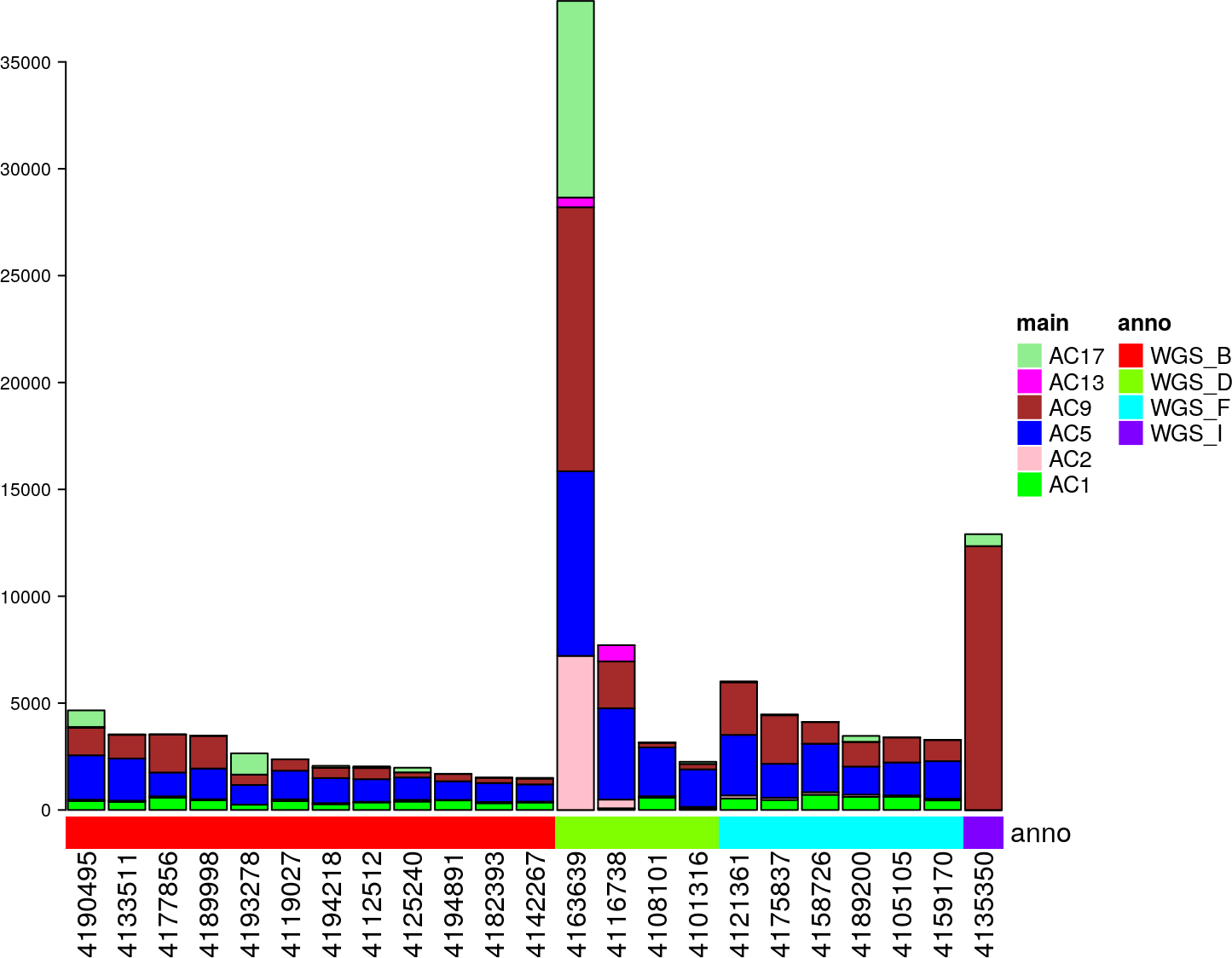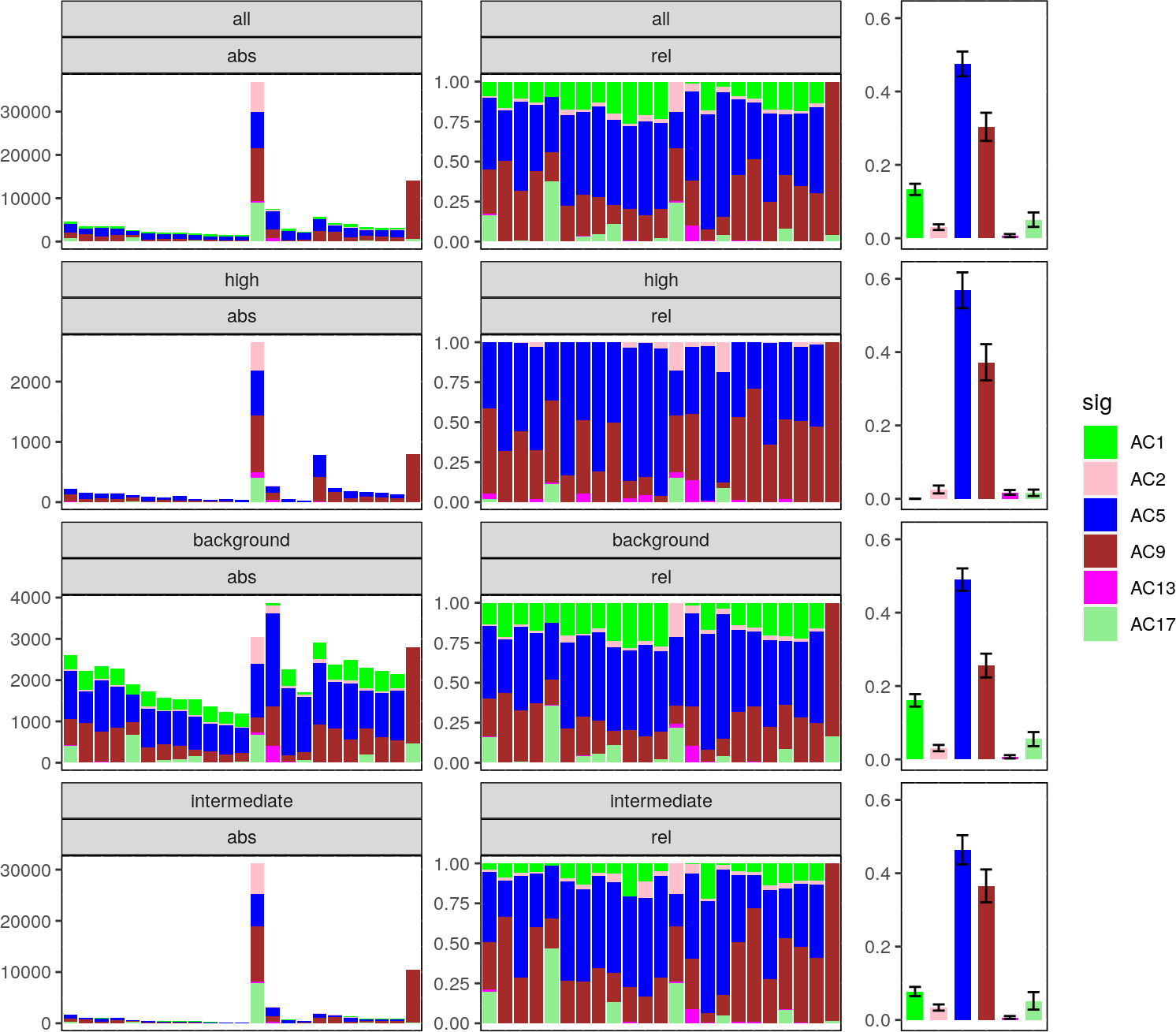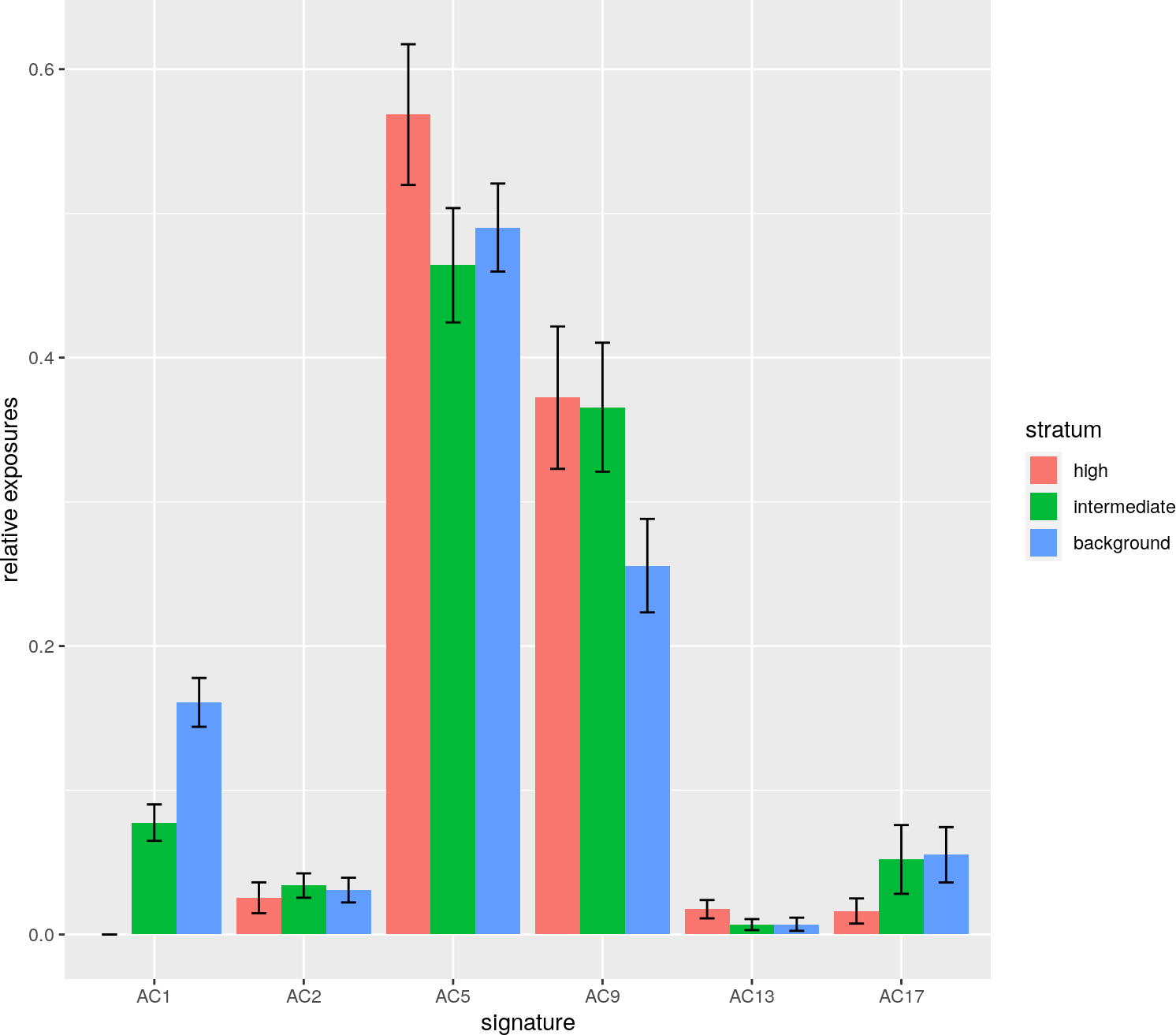# 1 Introduction

For many biological questions, it is of relevance to group mutations, or more specifically, single nucleotide variants (SNVs), into different strata or categories. These strata may be defined by genomic coordinates or other measures derived therefrom and annotated to the variants, e.g., replication timing, chromatin state, coding vs. non-coding part of the genome etc. Other choices of stratification are also possible, as shown below for the example of mutation density as stratification axis.

It is noteworthy to say that it would be neither sufficient nor appropriate to perform completely separate and independent NNLS analyses of mutational signatures on the different strata. This artificially reduces the statistical power provided by the higher number of SNVs in the unstratified setting. Instead, the results of the unstratified analysis should be used as input and supplied to a constrained analysis for the strata.

In YAPSA, these strata or categories have to be exclusive, i.e. one SNV must be in one and only one category. The number of strata will be denoted by $$s$$. For example one could evaluate whether an SNV falls into a region of high, intermediate or low mutation density by applying meaningful cutoffs on intermutation distance. Following this choice, there are three strata: high, intermediate and low mutation density. If we have already performed an analysis of mutational signatures for the whole (unstratified) mutational catalog of the cohort, we have identified a set of signatures of interest and the corresponding exposures. We now could ask the question if some of the signatures are enriched or depleted in one or the other stratum, yielding a strata-specific signature enrichment and depletion pattern. In YAPSA, the function SMC (Stratification of the Mutational Catalogue) solves the stratified optimization problem:

1. \begin{aligned} \min_{H_{(\cdot j)}^k \in \mathbb{R}^l}||W \cdot H_{(\cdot j)}^{k} - V_{(\cdot j)}^{k}|| \quad \forall j,k \\ \textrm{under the constraint of non-negativity:} \quad H_{(ij)}^{k} >= 0 \quad \forall i,j,k \\ \textrm{and the additional contraint:} \quad \sum_{k=1}^{s} H^{k} = H \\ \textrm{where H is defined by the optimization:} \quad \min_{H_{(\cdot j)} \in \mathbb{R}^l}||W \cdot H_{(\cdot j)} - V_{(\cdot j)}|| \quad \forall j \\ \textrm{also under the constraint of non-negativity:} \quad H_{(ij)} >= 0 \quad \forall i,j \quad \textrm{and} \quad V = \sum_{k=1}^{s} V^{k} \end{aligned}

As defined in 1. Usage of YAPSA, $$j$$ is the index over samples, $$m$$ is the number of samples, $$i$$ is the index over signatures and $$l$$ is the number of signatures. In addition, $$k$$ is the index over strata and $$s$$ is the number of strata. Note that the last two lines of equation (1) correspond to the unstratified optimization problem. The very last part of equation (1) reflects the additivity of the stratified mutational catalogs $$V^{k}$$ which is due to the fact that by definition the sets of SNVs they were constructed from (i.e. the strata) are exclusive.

The SMC-procedure can also be applied when an NMF analysis has been performed and the exposures $$\widetilde{H}$$ of this NMF analysis should be used as input and constraint for the SMC. It then solves the task:

1. \begin{aligned} \min_{H_{(\cdot j)}^k \in \mathbb{R}^l}||W \cdot H_{(\cdot j)}^{k} - V_{(\cdot j)}^{k}|| \quad \forall j,k \\ \textrm{under the constraint of non-negativity:} \quad H_{(ij)}^{k} >= 0 \quad \forall i,j,k \\ \textrm{and the additional contraint:} \quad \sum_{k=1}^{s} H^{k} = \widetilde{H} \\ \end{aligned}

Applying SMC that way, the initial LCD decomposition of the unstratified mutational catalog is omitted and it’s result replaced by the exposures extracted by the NMF analysis.

# 2 Recap: compute signature exposures (without stratification)

In the following section, we briefly recapitulate the analysis of SNV mutational signatures on an example data set as performed in 1. Usage of YAPSA. We thus first load the example data stored in the package:

data(sigs)
data(cutoffs)
data("lymphomaNature2013_mutCat_df")
current_cutoff_vector <- cutoffCosmicValid_abs_df[6,]

We then perform a supervised analysis of SNV mutational signatures using signature-specific cutoffs:

lymphoma_COSMIC_listsList <-
LCD_complex_cutoff_combined(
in_mutation_catalogue_df = lymphomaNature2013_mutCat_df,
in_cutoff_vector = current_cutoff_vector,
in_signatures_df = AlexCosmicValid_sig_df,
in_sig_ind_df = AlexCosmicValid_sigInd_df)

We assign subgroups to the different samples:

data(lymphoma_PID)
colnames(lymphoma_PID_df) <- "SUBGROUP"
lymphoma_PID_df$PID <- rownames(lymphoma_PID_df) COSMIC_subgroups_df <- make_subgroups_df(lymphoma_PID_df, lymphoma_COSMIC_listsList$cohort$exposures) And finally plot the obtained result: exposures_barplot( in_exposures_df = lymphoma_COSMIC_listsList$cohort$exposures, in_signatures_ind_df = lymphoma_COSMIC_listsList$cohort$out_sig_ind_df, in_subgroups_df = COSMIC_subgroups_df)Figure 1: Exposures to SNV mutational signatures # 3 Stratified analysis of mutational signatures ## 3.1 Preparation The functions for the stratified analysis access the raw data in order to provide the highest degree of flexibility for the definition of the strata themselves. Therefore we have to repeat the formatting steps already performed in the first vignette. data("lymphoma_Nature2013_raw") names(lymphoma_PID_df) <- gsub("SUBGROUP", "subgroup", names(lymphoma_PID_df)) names(lymphoma_Nature2013_raw_df) <- c("PID","TYPE","CHROM","START", "STOP","REF","ALT","FLAG") lymphoma_Nature2013_df <- subset(lymphoma_Nature2013_raw_df,TYPE=="subs", select=c(CHROM,START,REF,ALT,PID)) names(lymphoma_Nature2013_df) <- "POS" lymphoma_Nature2013_df$SUBGROUP <- "unknown"
DLBCL_ind <- grep("^DLBCL.*",lymphoma_Nature2013_df$PID) lymphoma_Nature2013_df$SUBGROUP[DLBCL_ind] <- "DLBCL_other"
MMML_ind <- grep("^41[0-9]+$",lymphoma_Nature2013_df$PID)
lymphoma_Nature2013_df <- lymphoma_Nature2013_df[MMML_ind,]
for(my_PID in rownames(lymphoma_PID_df)) {
PID_ind <- which(as.character(lymphoma_Nature2013_df$PID)==my_PID) lymphoma_Nature2013_df$SUBGROUP[PID_ind] <-
lymphoma_PID_df$subgroup[which(rownames(lymphoma_PID_df)==my_PID)] } lymphoma_Nature2013_df$SUBGROUP <- factor(lymphoma_Nature2013_df$SUBGROUP) lymphoma_Nature2013_df <- translate_to_hg19(lymphoma_Nature2013_df,"CHROM") lymphoma_Nature2013_df$change <-
attribute_nucleotide_exchanges(lymphoma_Nature2013_df)
lymphoma_Nature2013_df <-
lymphoma_Nature2013_df[order(lymphoma_Nature2013_df$PID, lymphoma_Nature2013_df$CHROM,
lymphoma_Nature2013_df$POS),] lymphoma_Nature2013_df <- annotate_intermut_dist_cohort(lymphoma_Nature2013_df, in_PID.field="PID") data("exchange_colour_vector") lymphoma_Nature2013_df$col <-
exchange_colour_vector[lymphoma_Nature2013_df$change] ## 3.2 Performing a stratification based on mutation density We will now use the intermutational distances computed above. We set cutoffs for the intermutational distance at 1000 and 100000 bp, leading to three strata. We annotate to every variant in which stratum it falls. lymphoma_Nature2013_df$density_cat <- cut(lymphoma_Nature2013_df$dist, c(0,1001,100001,Inf), right=FALSE, labels=c("high","intermediate", "background")) The following table shows the distribution of variants over strata: temp_df <- data.frame(table(lymphoma_Nature2013_df$density_cat))
names(temp_df) <- c("Stratum","Cohort-wide counts")
kable(temp_df, caption=paste0("Strata for the SMC of mutation density"))

Table 1: Strata for the SMC of mutation density
Stratum Cohort-wide counts
high 6818
intermediate 62131
background 50675

We now have everything at hand to carry out a stratified signature analysis:

strata_order_ind <- c(1,3,2)
mut_density_list <- run_SMC(lymphoma_Nature2013_df,
lymphoma_COSMIC_listsList$cohort$signatures,
lymphoma_COSMIC_listsList$cohort$out_sig_ind_df,
COSMIC_subgroups_df,
column_name="density_cat",
refGenome=BSgenome.Hsapiens.UCSC.hg19,
cohort_method_flag="norm_PIDs",
in_strata_order_ind=strata_order_ind)Figure 2: SMC (Stratification of the Mutational Catalogue)
based on mutation density.

This produces a multi-panel figure with 4 rows of plots. The first row visualizes the signature distribution over the whole cohort without stratification, followed by one row of plots per stratum. Hence in our example we have four rows of graphs with three (exclusive) strata as input. Each row consists of three plots. The left plots show absolute exposures in the respective stratum as stacked barplots on a per sample basis. The middle plots show relative exposures in the respective stratum on a per sample basis as stacked barplots. The right plots shows cohort-wide averages of the relative exposures in the respective stratum. The error bars indicate the standard error of the mean (SEM).

These results can also be displayed as a dodged barplot containing the information of the third column of plots in the above figure, which needs the execution of an additional code block for its generation:

dodged_df <- do.call(rbind, mut_density_list$cohort) names(dodged_df) <- gsub("variable","stratum", names(dodged_df)) names(dodged_df) <- gsub("sig","signature", names(dodged_df)) dodged_df$stratum <- gsub("_rel", "", as.character(dodged_df$stratum)) dodged_df <- dodged_df[which(dodged_df$stratum != "all"),]
dodged_df$stratum <- factor(as.character(dodged_df$stratum),
levels = sort(unique(dodged_df$stratum))[rev(strata_order_ind)]) dodged_plot <- ggplot() + geom_bar(data = dodged_df, aes_string(x = "signature", y = "exposure", group = "stratum", fill = "stratum"), stat = "identity", position = "dodge", size = 1.5) + geom_errorbar(data = dodged_df, aes_string(x = "signature", ymin = "exposure_min", ymax = "exposure_max", group = "stratum"), position = position_dodge(width = 0.9), width = 0.3) + labs(y = "relative exposures") if(exists("current_strata_colVector")){ dodged_plot <- dodged_plot + scale_fill_manual(values = current_strata_colVector[-1], labels = current_labelVector[-1] [current_strata_order_ind]) } print(dodged_plot)Figure 3: SMC results displayed as dodged plot ## 3.3 Testing for statistical significance of enrichment and depletion patterns To test for statistical significance of potential differences in the signature exposures (i.e. signature enrichment and depletion patterns) between the different strata, we can use the Kruskal-Wallis test, as the data is grouped into (potentially more than two) categories and might not follow a normal distribution. As we are testing the different signatures on the same stratification, we have to correct for multiple testing. In order to control the false discovery rate (FDR), the Benjamini-Hochberg correction is appropriate. stat_mut_density_list <- stat_test_SMC(mut_density_list,in_flag="norm") kable(stat_mut_density_list$kruskal_df,
caption=paste0("Results of Kruskal tests for cohort-wide exposures over",
" strata per signature without and with correction for ",
"multiple testing."))

Table 2: Results of Kruskal tests for cohort-wide exposures over strata per signature without and with correction for multiple testing.
Kruskal_statistic df Kruskal_p_val Kruskal_p_val_BH
AC1 45.762664 2 0.0000000 0.0000000
AC2 5.612044 2 0.0604450 0.1006065
AC5 1.964605 2 0.3744480 0.4493376
AC9 6.068377 2 0.0481137 0.1006065
AC13 1.427930 2 0.4896988 0.4896988
AC17 5.404008 2 0.0670710 0.1006065

In the following paragraph we perform post-hoc tests for those signatures where the Kruskal-Wallis test, as evaluated above, has given a significant result.

significance_level <- 0.05
for(i in seq_len(dim(stat_mut_density_list$kruskal_df))){ if(stat_mut_density_list$kruskal_df$Kruskal_p_val_BH[i]<significance_level){ print(paste0("Signature: ",rownames(stat_mut_density_list$kruskal_df)[i]))
print(stat_mut_density_list\$kruskal_posthoc_list[[i]])
}
}
##  "Signature: AC1"
##
##  Pairwise comparisons using Tukey and Kramer (Nemenyi) test
##                    with Tukey-Dist approximation for independent samples
##
## data:  sig_exposures_vector and sig_strata_vector
##
##              background high
## high         3.0e-10    -
## intermediate 0.058      8.6e-05
##
## P value adjustment method: none

From this analysis, we can see that a distinct signature enrichment and depletion pattern emerges:

1. Stratum of high mutation density: Enrichment of signatures AC5 and depletion of signatures AC1 (significant) and AC17
2. Background: signature distribution very similar to the one of the complete mutational catalog (first row)
3. Stratum of intermediate mutation density: intermediate signature enrichment and depletion pattern between the strata of high mutation density and background.